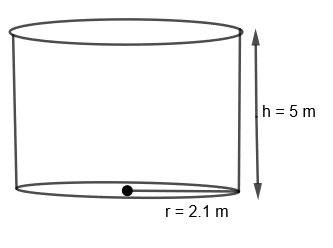QUESTION

# Find the capacity of the cylindrical water tank whose radius is 2.1 m and length is 5m.

Hint: First of all try to recollect what the capacity of an object means. Now find the capacity of the cylindrical tank by using the formula $V=\pi {{r}^{2}}h$ where r and h are the radius and height of the tank respectively.

In this question, we have to find the capacity of the cylindrical water tank whose radius is 2.1 m and length/height is 5m. Before proceeding with this question, we must know the meaning of the capacity of any object. Capacity is nothing but the amount that some object can hold or in other words, we can say that it is the volume of an object. Volume is the quantity that all three-dimensional objects have. It is the space occupied by the object. Volume is measured in cubic units like ${{m}^{3}},c{{m}^{3}}$, etc, or in liters, milliliters, etc. If we have two containers, one has a capacity of 5 liters and the second has a capacity of 10 liters. Then, we can say that the volume of the second container is more than the first container.We know that the volume of the cylinder is $\pi {{r}^{2}}h$ where r is the radius of the cylinder and h is the height of the cylinder. So, as we also get the volume of the cylindrical tank = $\pi {{r}^{2}}h$
The volume of the cylindrical tank $=\pi {{\left( 2.1 \right)}^{2}}.5\text{ }{{m}^{3}}$.
By substituting the value of $\pi =\dfrac{22}{7}$, we get,
The volume of the cylindrical tank $=\dfrac{22}{7}\times \left( 2.1 \right)\times \left( 2.1 \right)\times 5=69.3\text{ }{{m}^{3}}$
Note: Here, students must note that whenever we are asked to find the capacity of any object or vessel, we are indirectly asked to find the volume of that object. Also, we must remember the formulas of the volumes some regulate solids like cubes, cuboids, cylinders, sphere, etc. Also, many students make this mistake of writing units of volume incorrectly, that is they write the volume in ${{m}^{2}},c{{m}^{2}},etc.$ which is wrong. The volume of an object is always in cubic units like ${{m}^{3}},c{{m}^{3}},etc.$ as it takes all three dimensions of the object distance to find the volume.